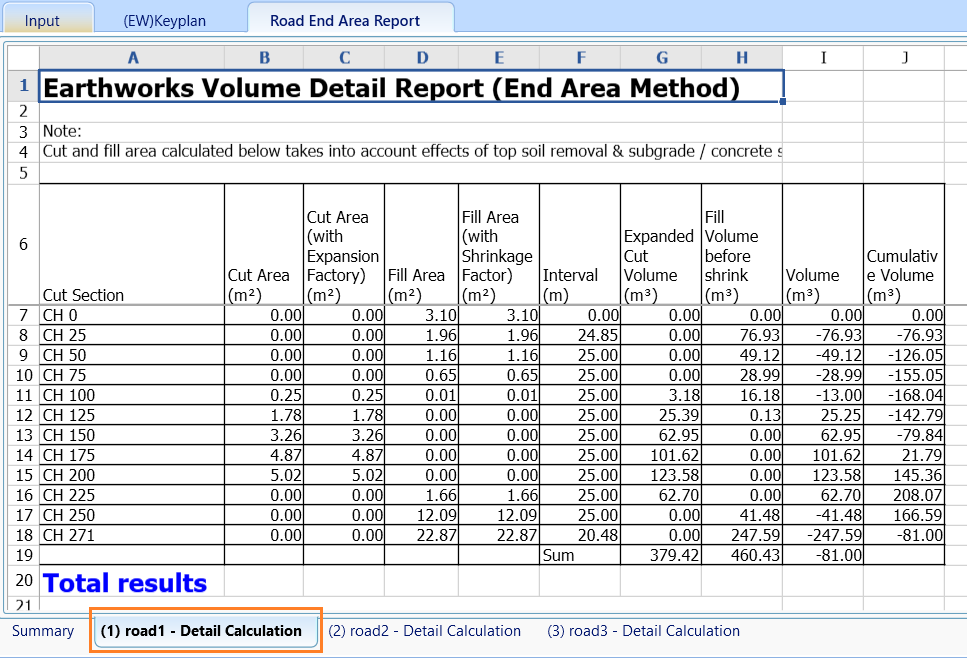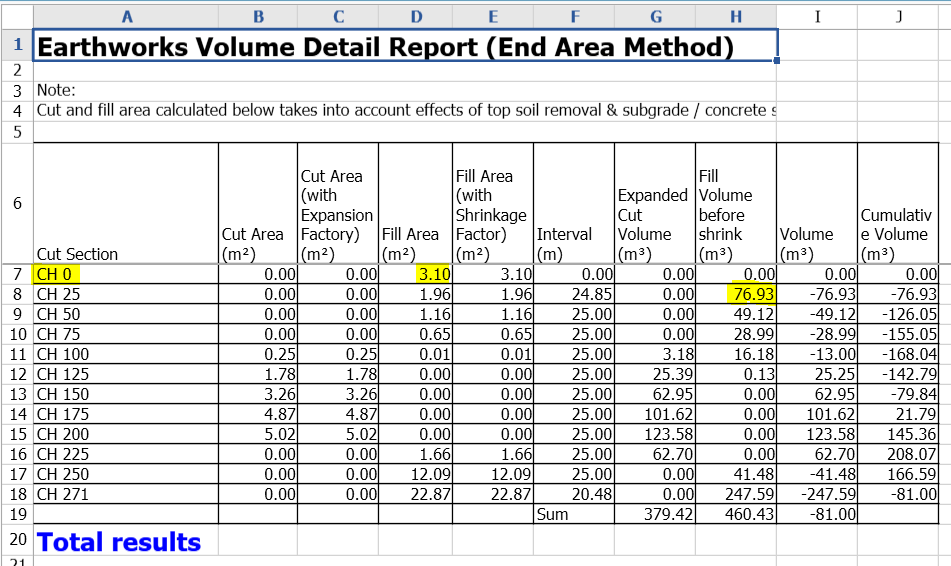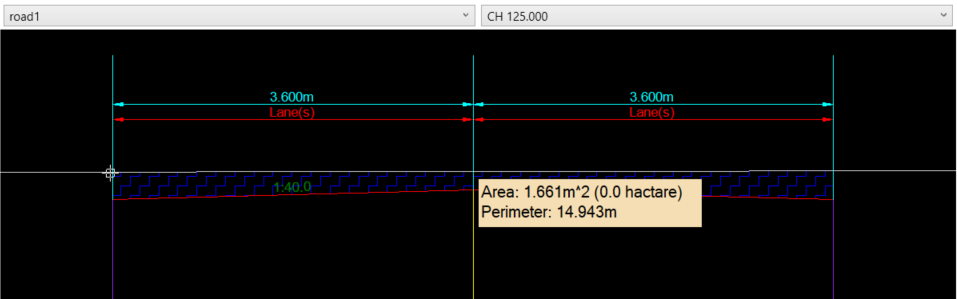603 - 5885 1250 (4 lines) info@mes100.com
Select Page

Version use: 2.5.36.0

Project file here

In MiTS 2, the cut fill volume can be calculated by the ‘End Area’ method.The road end area calculation is calculated per road basis. The cut and fill volume is calculated in a way that the other platforms or road will not be included.

(Calculation is done as if there are no other platforms/roads

As per image below, the calculation shown is as per road basis.The basis of earthwork volume will be dependent upon the road cross section as the road cross section solely depending upon the parameter set is the parameter dialog.

If the slope is set to YES, hence the cut and fill volume will include the slope area and if NO then the cut and fill volume will exclude the slope area.a) With slope

i.e; CH 0Cross section CH 0

Volume of fill = Fill area x chainage interval

ஃ Volume of fill = 3.096m2 x 24.85m

= 76.93mb) Without slope

i.e; CH 125Cross section CH 125Cross section CH 150

Volume of cut = (Cut area at CH 125 = Cut area at CH 150)/2 x chainage interval

ஃ Volume of cut = (1.661m2 + 2.947m2)/2 x 25m

= 57.60mNote:

• The cut and fill volume is solely dependent on the road cross section
• While the road cross section slope is solely depending on a parameter in the parameter dialog